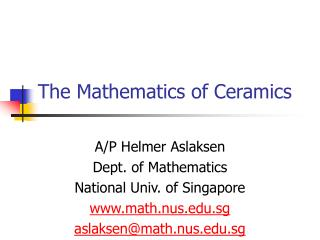# The Mathematics of Ceramics - PowerPoint PPT PresentationDownload PresentationThe Mathematics of Ceramics

The Mathematics of Ceramics
Download Presentation## The Mathematics of Ceramics

- - - - - - - - - - - - - - - - - - - - - - - - - - - E N D - - - - - - - - - - - - - - - - - - - - - - - - - - -
##### Presentation Transcript

1. The Mathematics of Ceramics A/P Helmer Aslaksen Dept. of Mathematics National Univ. of Singapore www.math.nus.edu.sg aslaksen@math.nus.edu.sg

2. What does math have to do with ceramics? • What is math? • Math is the abstract study of patterns • What is a pattern? • Concrete geometrical patterns or abstract numerical or logical patterns • What is abstract study? • Generalize to get the underlying concept

3. Where in Singapore is this?

4. Why are these patterns nice? • Symmetry • What is symmetry? • Most people think of vertical mirror symmetry (left/right)

5. What is symmetry in general? • A pattern is symmetric if it is built up from related parts • A plane pattern has a symmetry if there is an isometry of the plane that preserves the pattern

6. An isometry of the plane is a mapping that preserves distance, and therefore shape What is an isometry?

7. Rotation • A rotation has a centre of rotation and an angle of rotation

8. N-fold rotation • If the angle is θ and n = 360o/θ is a whole number, then we call the rotation an n-fold rotation

9. Rotational symmetry

10. Glide reflection • A glide reflection is a combination of a reflection and a translation

11. Four types of isometries • Translation • Reflections • Rotations • Glide reflections

12. Symmetric patterns • A plane pattern has a symmetry if there is an isometry of the plane that preserves it. There are three types of symmetric patterns. • Rosette patterns (finite designs) • Frieze patterns • Wallpaper patterns

13. Rosette patterns • Leonardo’s Theorem: There are two types of rosette patterns. • Cn, which has n-fold rotational symmetry and no reflectional symmetry • Dn, which has n-fold rotational symmetry and reflectional symmetry

14. Examples of rosette patterns

15. Frieze patterns • Frieze patterns are patterns that have translational symmetry in one direction • We imagine that they go on to infinity in both directions or wrap around

16. Frieze Patterns

17. Examples of frieze patterns • No sym LLLL • Half turn NNN • Hor ref DDD • Ver ref VVV • Glide ref • Hor and ver ref HHH • Glide ref and ver ref

18. Wallpaper • There are 17 types of wall paper patterns

19. What does this have to do with arts? • Every culture has a preference for certain symmetry type of patterns. • The important thing is not the motif in the patterns, but the symmetry types. • This can be used to date objects and detect connections between different cultures.

20. Ming ceramics • We will study Ming ceramics as an example

21. No symmetry • The p111 pattern (no symmetry)

22. Horizontal reflection • The p1m1 pattern (horizontal reflection)

23. Vertical reflection • The pm11 pattern (vertical reflection)

24. Half turn • The p112 pattern (half turn)

25. Horizontal and vertical reflection • The pmm2 pattern (horizontal and vertical reflections)

26. Glide reflection and vertical reflection • The pma2 pattern (glide reflection and vertical reflection)

27. Glide reflection • The p1a1 pattern (glide reflection)

28. Analysis-Ming Porcelains

29. Analysis-Ming Porcelains

30. Analysis-Ming Porcelains

31. Analysis-Ming Porcelains

32. Analysis-Ming Porcelains

33. Analysis-Ming Porcelains

34. Peranakan Ceramics • We also looked at the Peranakan ceramics at the Asian Civilisations Museum in Singapore

35. No symmetry • The p111 pattern

36. Vertical reflection • The pm11 pattern

37. Half turn • The p112 pattern

38. Horizontal and vertical reflection • The pmm2 pattern

39. Glide reflection and vertical reflection • The pma2 pattern pma2 pm11

40. Glide reflection • The p1a1 pattern

41. Analysis-Peranakan Porcelains

42. Analysis-Peranakan Porcelains

43. Analysis- Peranakan Porcelains